# Perform math operations on a range of cells

Using the Calculate tool, you can apply basic mathematical operations, such as addition, multiplication, subtraction, or division, to the entire range in a click. What's more, you can add and subtract percentages or find the percentage of a number - all for multiple cells at once.

## How to do quick calculations in Excel

1. Select a range that contains the numbers to calculate in your Excel table: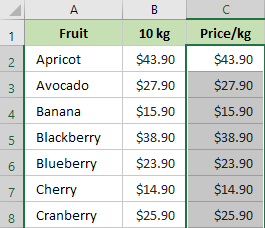2. On the Ablebits Tools tab, in the Calculate group, click the arrow in the Operation dropdown box and select the operation you need to perform. In the Value box, enter a number: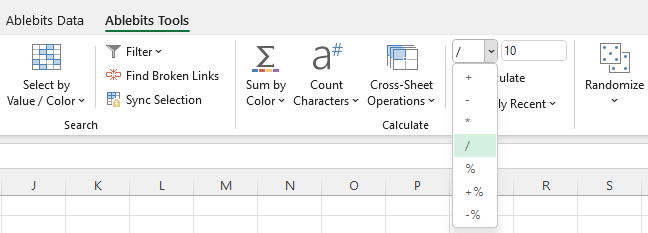3. Click Calculate: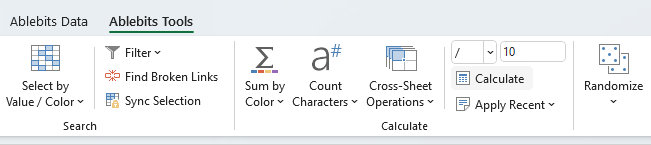And see the result: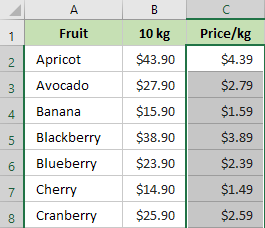Note. If the cell/range in your table contains a formula, it will be modified according to the operation you select. For example, you have a formula "=A1+B1" in the selected cell and you choose to calculate the percentage "- %50". When you click Calculate, the formula will change to "=(A1+B1)*0.5". In other words, this changes whole formulas, not only values.
Tip. The last 10 operations are saved in the Apply Recent list. To see the latest operations, click the Apply Recent button and pick the operation you'd like to apply to the selected cell or range.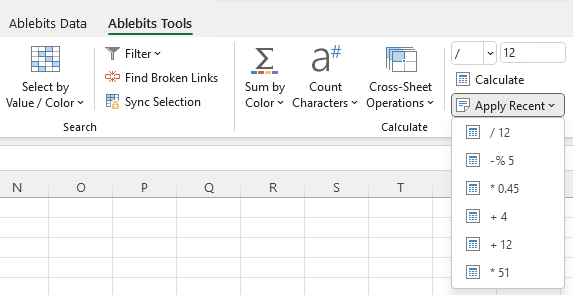ResponsesHenri van Aswegen says:
July 28, 2020 at 10:41 am

Can you assist. I have column D that indicates the number of animals, 20 species, column F has the price of the animals and column E the discount percentage. Can you help me with a formula where the total of column D determines the percentage of discount. i.e. if the total is three animals, it automatically shows a 5% discount in column E, 7 animals 10% and 10 animals total 15%.Hello Henri,

Thank you for your message. Sorry, we do not provide support for generic Excel issues and questions. However, you may find plenty of videos and how-to tutorials on our blog. I believe you'll find the solution in one of the articles there. Otherwise, please ask your question in the Comments section under the corresponding topic and our tech specialist will do his best to help you out. Thank you.Kevin Mathews says:
November 8, 2019 at 10:20 pm

Is it possible to revise a range of formulas? For example, apply Round([existing formula],1).Katerina Bespalaya (Ablebits Team) says:
November 11, 2019 at 11:28 am

Hello Kevin,

Unfortunately, we don't have a tool in our Ultimate Suite for Excel that could perform this operation.I need to calculate the difference between a start time and end time in minutes. Always on the same day so the date is not necessary. How would I do this?Hello Lisa,
Thank you for contacting us.

It looks like our Date & Time Formula Wizard may come in handy. It allows you to find a difference between two times in a few clicks. This add-in is available as a part of our Ultimate Suite for Excel that you can install in a trial mode and use it for 30 days for free. Here is the direct download link for you: https://www.ablebits.com/files/get.php?addin=xl-suite&f=free-trial

You can also check the detailed instructions on how to work with Date & Time Formula Wizard on its help page: https://www.ablebits.com/docs/excel-calculate-dates-time-age/Good Day

Hope this message finds you well...

I have this arithmetic Patten that just adds a certain number,I used the =SUM function to get my value which was correct, but to continue with the Patten I need to drag it down to my desired value without reputation of the initial answer.

The patten goes like:

1234
1345
1456 and so on...

to get that I used the difference of each number, which is 111. But now I need excel to automatically add this 111 to the answer I get when dragging down the column to get the Patten straight without reputation .
I hope my explanation is clear, your assistance will be highly appreciated.

Regards

TshepangHi Tshepang,
If I have understood your task correctly, the solution is simple. You don’t even have to use the SUM function.
So,
1. Enter 1234 into A1.
2. 1345 into A2.
3. 1456 into A3.
4. Select the A1:A3 range and you will see the fill handle that is a little square in the lower-right corner of the selection.
5. Click on the fill handle and drag it down. The cells will be automatically filled with the numbers you need:
1234
1345
1456
1567
1678
1789
1900